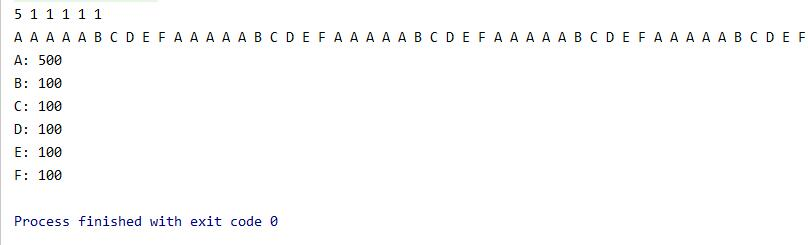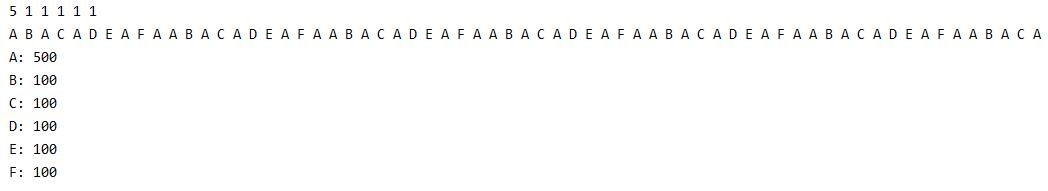# 分布式系统：负载均衡算法

## 带权重的轮询负载均衡算法

func doSimpleWeightBound(servers []Server,initWeight []int){
res:=list.New()
weight:=make([]int,len(initWeight))
count:=make([]int, len(weight))
totalWeight:=0
for i := 0; i< len(initWeight);i++  {
totalWeight+=initWeight[i]
}
copy(weight,initWeight)
nowWeight:=totalWeight
//一共做totalWeight*100次，查看结果。
for i := 0; i < totalWeight*100; i++ {
//1. 选出当前权重最高的机器
position:=selectHighWeight(weight)
count[position]++
res.PushBack(servers[position].name)
//2. 选出的机器权重数减1
weight[position]--
//3. 总权重减1
nowWeight--
if(nowWeight<=0){
copy(weight,initWeight)
nowWeight=totalWeight
}
}
for i :=0; i < len(weight); i ++ {
fmt.Printf("%d ",weight[i])
}
fmt.Println()
for i := res.Front(); i != nil; i = i.Next() {
fmt.Printf("%s ",i.Value)
}
fmt.Println()
for i :=0; i < len(count); i ++ {
fmt.Printf("%s: %d\n",string('A'+i),count[i])
}
}## 平滑带权重的轮询负载均衡算法

1. 建立两个数组，initWeight记录初始各机器的权重，weight记录过程中的权重。
2. 求得初始总权重totalWeight。
3. 从各机器中选出当前权重weight最高的一台机器S1。
4. S1对应的当前权重减去总权重totalWeight。
5. 每台机器的当前权重weight[i]依次增加各机器初始的权重initWeight[i]
6. 重复3-5步骤。
func doSmoothlyWeightBound(servers []Server,initWeight []int){
//一共做30次，分别看选出的是哪台机器。
res:=list.New()
weight:=make([]int,len(initWeight))
count:=make([]int, len(weight))
totalWeight:=0
for i := 0; i< len(initWeight);i++  {
totalWeight+=initWeight[i]
}
copy(weight,initWeight)
for i := 0; i < totalWeight*8; i++ {
//1. 选出当前权重最高的机器
position:=selectHighWeight(weight)
count[position]++
res.PushBack(servers[position].name)
//2. 选出的机器减去总权重
weight[position]-=totalWeight
//3. 对每台机器，增加初始权重
for j := 0; j < len(weight); j++ {
weight[j]+=initWeight[j]
}
}
for i :=0; i < len(weight); i ++ {
fmt.Printf("%d ",weight[i])
}
fmt.Println()
for i := res.Front(); i != nil; i = i.Next() {
fmt.Printf("%s ",i.Value)
}
fmt.Println()
for i :=0; i < len(count); i ++ {
fmt.Printf("%s: %d\n",string('A'+i),count[i])
}
}## 总结

posted @ 2020-10-14 15:30  IntoTw  阅读(442)  评论(0编辑  收藏  举报